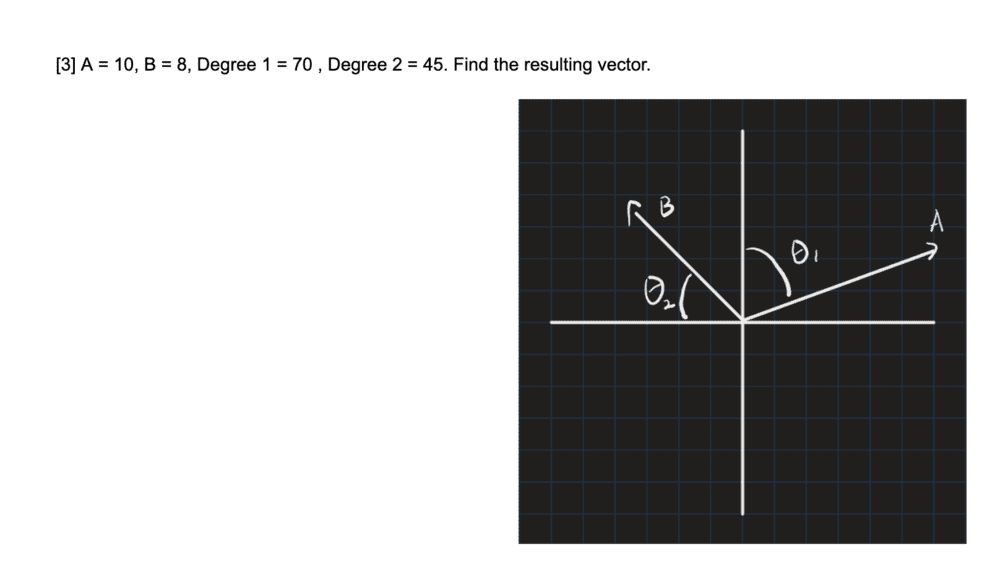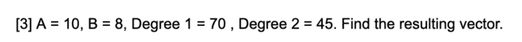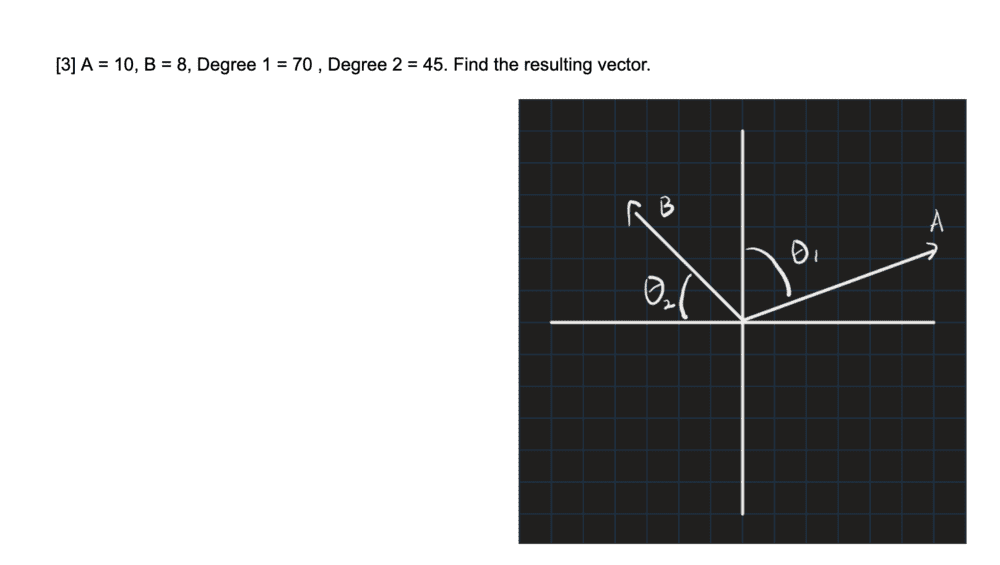# Vector Problem -- Addition of two vectors given in polar coordinates

randomphysicsguy123
Homework Statement:
Find the resulting vector
Relevant Equations:
c^2=a^2+b^2
Doing a review for my SAT Physics test and I'm practicing vectors. However, I am lost on this problem I know I need to use trigonometry to get the lengths then use c^2=a^2+b^2. But I need help going about this.Last edited by a moderator:

Homework Helper
Gold Member
2022 Award
What do you know about vector components?

Note that you have to make an effort yourself.

randomphysicsguy123
I have made an effort however I am unsure if my first step of 10sin(70) and 8tan(45) is correct as I do not want to go off track from the start

Homework Helper
Gold Member
2022 Award
I have made an effort however I am unsure if my first step of 10sin(70) and 8tan(45) is correct as I do not want to go off track from the start
It's hardly a long, extended problem. You have to post what you've done.

Note that ##10\sin(70)## by itself is just a number. What do you intend to do with that number?

randomphysicsguy123
Work:
x=10cos70=3.4

x=8cos45=5.65

y=10sin70=9.3

y=8sin45=5.65

x(hat)=3.4+5.65=9.05

y(hat)=9.3+5.65=14.95

R=sqrt(9.05^2+14.95^2)=17.47

R=17.47

Mentor
Work:
x=10cos70=3.4

x=8cos45=5.65

y=10sin70=9.3

y=8sin45=5.65

x(hat)=3.4+5.65=9.05

y(hat)=9.3+5.65=14.95

R=sqrt(9.05^2+14.95^2)=17.47

R=17.47
I'll try to look through that in a bit, but a couple of suggestions:

** Please check out the LaTeX Guide at the lower left of the Edit window. That's the best way to post math equations at the PF and many other websites

** It would help if you commented each line that you posted. Presumably you are resolving the two vectors into rectangular coordinates and adding those components. Is that what you are doing?

randomphysicsguy123
My bad I will comment on my lines in the future as for what I am doing I wanted to sum the two vectors, so I first needed to decompose both vectors into their component form. Then, I added these components together and then used the Pythagorean theorem to find the magnitude.

•berkeman
MentorYour image does not seem to match your problem statement. Why have you labeled ##\theta_1## down from the y-axis? Both angles should be referenced to the positive x-axis to get the signs and magnitudes right. And you are correct that you need to add the x and y components of each vector in rectangular coordinates to figure out the overall sum vector...

randomphysicsguy123
This image was the one provided.

Last edited by a moderator:
randomphysicsguy123
It is not from an SAT prep book, it is from a free online SAT Physics site that provides example questions. Thus this is the real question. I am simply asking if I did it right and if not what did I do wrong and how to correct it.

Mentor
It is not from an SAT prep book, it is from a free online SAT Physics site that provides example questions. Thus this is the real question. I am simply asking if I did it right and if not what did I do wrong and how to correct it.
Fair enough. Is that the image that they provided, or your initial attempt at drawing it? I'm not trying to give you a hard time, I just need to understand what parts to translate into LaTeX for you as and example and to verify your work.

Can you please post a link to the original problem so that there is no more ambiguity? Thank you.